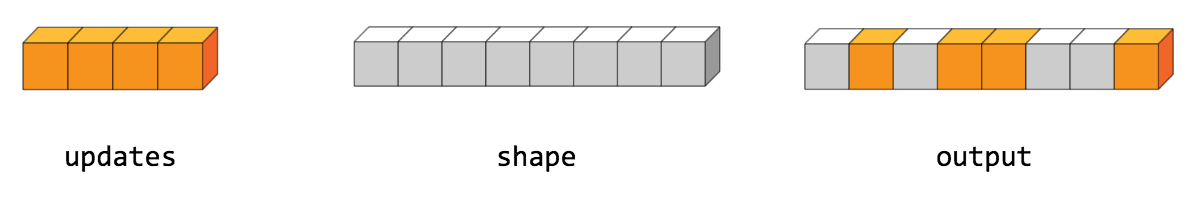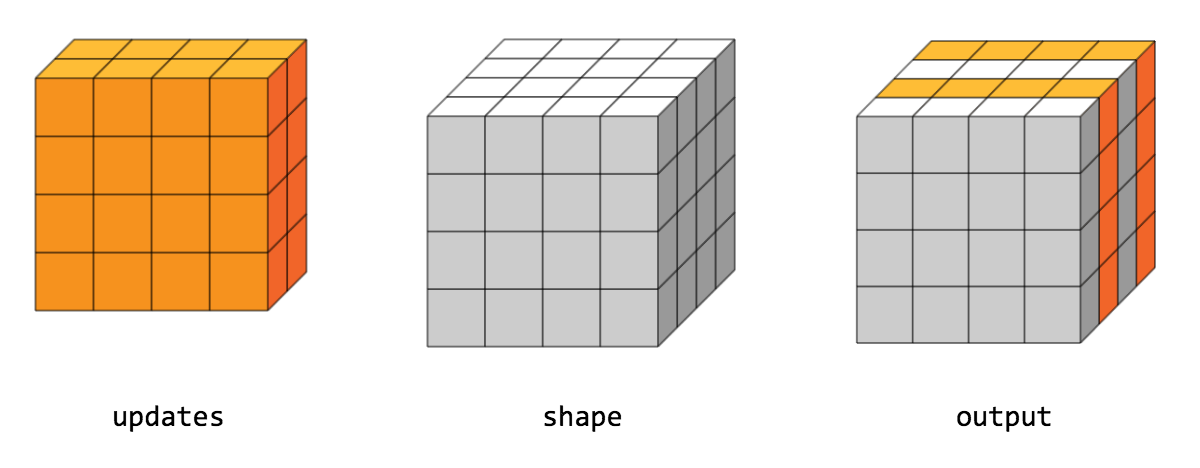Help protect the Great Barrier Reef with TensorFlow on Kaggle

tf.scatter_nd

Scatter updates into a new tensor according to indices.

Creates a new tensor by applying sparse updates to individual values or slices within a tensor (initially zero for numeric, empty for string) of the given shape according to indices. This operator is the inverse of the tf.gather_nd operator which extracts values or slices from a given tensor.

This operation is similar to tensor_scatter_add, except that the tensor is zero-initialized. Calling tf.scatter_nd(indices, values, shape) is identical to tensor_scatter_add(tf.zeros(shape, values.dtype), indices, values)

If indices contains duplicates, then their updates are accumulated (summed).

indices is an integer tensor containing indices into a new tensor of shape shape. The last dimension of indices can be at most the rank of shape:

indices.shape[-1] <= shape.rank

The last dimension of indices corresponds to indices into elements (if indices.shape[-1] = shape.rank) or slices (if indices.shape[-1] < shape.rank) along dimension indices.shape[-1] of shape. updates is a tensor with shape

indices.shape[:-1] + shape[indices.shape[-1]:]

The simplest form of scatter is to insert individual elements in a tensor by index. For example, say we want to insert 4 scattered elements in a rank-1 tensor with 8 elements.In Python, this scatter operation would look like this:

indices = tf.constant([, , , ])
updates = tf.constant([9, 10, 11, 12])
shape = tf.constant()
print(scatter)

The resulting tensor would look like this:

[0, 11, 0, 10, 9, 0, 0, 12]

We can also, insert entire slices of a higher rank tensor all at once. For example, if we wanted to insert two slices in the first dimension of a rank-3 tensor with two matrices of new values.In Python, this scatter operation would look like this:

indices = tf.constant([, ])
updates = tf.constant([[[5, 5, 5, 5], [6, 6, 6, 6],
[7, 7, 7, 7], [8, 8, 8, 8]],
[[5, 5, 5, 5], [6, 6, 6, 6],
[7, 7, 7, 7], [8, 8, 8, 8]]])
shape = tf.constant([4, 4, 4])
print(scatter)

The resulting tensor would look like this:

[[[5, 5, 5, 5], [6, 6, 6, 6], [7, 7, 7, 7], [8, 8, 8, 8]],
[[0, 0, 0, 0], [0, 0, 0, 0], [0, 0, 0, 0], [0, 0, 0, 0]],
[[5, 5, 5, 5], [6, 6, 6, 6], [7, 7, 7, 7], [8, 8, 8, 8]],
[[0, 0, 0, 0], [0, 0, 0, 0], [0, 0, 0, 0], [0, 0, 0, 0]]]

Note that on CPU, if an out of bound index is found, an error is returned. On GPU, if an out of bound index is found, the index is ignored.

indices A Tensor. Must be one of the following types: int32, int64. Index tensor.